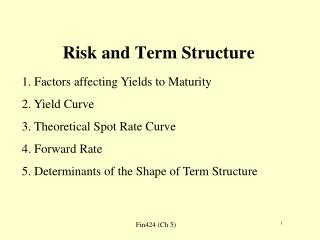DownloadDownload PresentationRisk and Term Structure

# Risk and Term Structure

Télécharger la présentation## Risk and Term Structure

- - - - - - - - - - - - - - - - - - - - - - - - - - - E N D - - - - - - - - - - - - - - - - - - - - - - - - - - -
##### Presentation Transcript

1. Risk and Term Structure 1. Factors affecting Yields to Maturity 2. Yield Curve 3. Theoretical Spot Rate Curve 4. Forward Rate 5. Determinants of the Shape of Term Structure

3. Understanding Yield to Maturity • We have two bonds, one bond coupon rate is 5%, the other is 0.5%. Which one is better?

4. Factors Affecting Bond Yield • Type of Issuers • Perceived credit worthiness of an issuer • Inclusion of options • Taxability of interest • Expected liquidity of an issue • Financeability of an issue • Term to maturity

5. Yield Curve • The graphic depiction of the relationship between yields of maturity on bonds of the same credit quality but different maturity • YTM of coupon bonds • See three shapes on page 100

6. Problem with Traditional Yield Curve • Securities with the same maturity may carry different yields since the timing of cash flows differ. • Thus it is hard to say anything about yield curve since the timing of cash flows is unknown.

7. Solution • Convert yield curve to theoretical spot rate curve. It is a graphic depiction of the term structure of interest rate. • Spot rate: the yield on a zero-coupon treasury with the same maturity • Theoretical spot rate

8. Constructing Theoretical Spot Rate Curve • Each cash flow should be discounted at a unique interest rate that is appropriate for the time period in which the cash flow will be received. • On-the-run treasury issues • On-the-run treasury and selected off-the-run treasury issue • All treasury coupon securities and bills • Treasure coupon strips

9. Constructing Theoretical Spot Rate Curve (pages 102-105) • On-the-run treasury issues: 3m, 6m, 1y, 2y, 5y, 10y, 30y treasury bonds • Get the theoretical spot rate curve. • Procedure • Get missing maturity points, obtain yield curve • Get theoretical spot rate

10. Problem with using just the on-the-run issues • Large gaps between maturities, particularly after 5 years

11. Linear Interpolation • A way to figure out yields of missing maturities • Difference in yields of high and low maturity/ • (# of semiannual periods + 1) • Page 102 and 103

12. Compute Theoretical Spot Rates • Page 104-106 • Trick: when yield=coupon rate, the bond is sold at par.

13. Stripped Treasury Securities Purchase treasury bonds and deposit them in a bank custody account Firms issue receipts representing an ownership interest in each coupon payment (coupon) and maturity value (corpus) of the underlying treasury bond Coupon stripping

14. Example: STRIP \$100 million face value Treasury bond with a 20-year maturity and a coupon of 10% is purchased to create zero-coupon treasury securities. (see pages 135, ch 6) How many bonds are created? Face values of different bonds? Maturity dates of the stripped bonds?

15. Forward Rate • The example on two equivalent bonds on page 109 • Formula for forward rate determination more than 2 periods: page 111

16. Determinants of Yield Curve Shape • (1) Pure expectation theory • forward rates exclusively represent the expected future spot rates: • f 1->2= E(z2) + l2 • Problem: ignore the risk regarding future interest rate, thus ignore the risk premium when investing in long-term bonds

17. (2) Liquidity Theory • The forward rate reflects both interest-rate expectation and a liquidity premium • f 1->2= E(z2) + l2 • Note: z2 is the 1-period rate at time 2, l2 is liquidity premium for a 2-period bond at time 1 (now) • That is, implied forward rate is no longer determined by previously mentioned formula. We should incorporate risk premiums • Even one expects the long-term interest rate to go down, E(z2)<z1, f could still be greater than z1

18. (3) Preferred Habitat Theory • Do all investors intend to liquidate their investment at the shortest possible date? • Interest rate is determined by investors’ preference. • Expectation plays no role under this theory, though the link between f and z1 and z2 still hold given no arbitrage between the forward rate and spot rates.

19. Market Segmentation Theory • The shape of yield curve is determined by the supply and supply for securities within each maturity sector. • Similar to the liquidity premium theory.